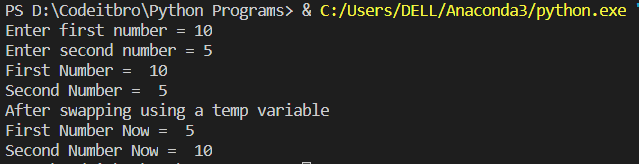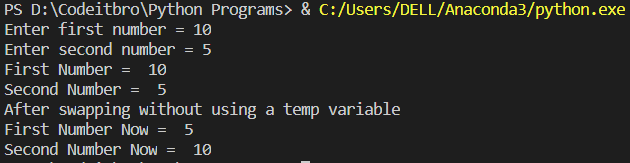# Python 3 Program To Swap Two Numbers

2436

Here you will find the Python 3 program to swap two numbers. Before getting started, you should know about basic Python concepts such as data types, variables, and operators. Also, check out these best Python books, if you want to learn Python more comprehensively.

## Python 3 program to swap two numbers using a third variable

Here, we will use a temporary variable (xyz) to swap two numbers (firstNum and secondNum). We will first store the value of firstNum in xyz and then save the value of secondNum in firstNum. At last, we will save the value of xyz to secondNum. In this way, two numbers are swapped using a temporary variable.

```firstNum = input("Enter first number = ")
secondNum = input("Enter second number = ")
print("First Number = ", firstNum)
print("Second Number = ", secondNum)
print("After swapping using a temp variable")

#code to swap two numbers using a third variable

xyz = firstNum
firstNum = secondNum
secondNum = xyz

print("First Number Now = ", firstNum)
print("Second Number Now = ", secondNum)```

## Output## Python 3 program to swap two numbers without using third variable

There is a simple construct to swap variables in Python. The code below does the same.

```firstNum = input("Enter first number = ")
secondNum = input("Enter second number = ")
print("First Number = ", firstNum)
print("Second Number = ", secondNum)

#Code to swap two numbers without any third variable

firstNum, secondNum = secondNum, firstNum
print("After swapping without using a temp variable")
print("First Number Now = ", firstNum)
print("Second Number Now = ", secondNum)```

## Output